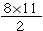# Surface Area

The surface area of a solid figure is the sum of the areas of all exposed sides of a figure. You can calculate the area of a figure by finding the areas of all of its faces and adding them. In sixth grade, you learned to calculate surface area using a net, or a two-dimensional representation of all sides of a solid. In this lesson, you will find the surface areas for right prisms and composed figures.

Let us start with basic shapes just as we did in the previous lesson.Use the formula S = 2lw +2lh+2wh and insert the dimensions

S = 2(7×7) + 2(7×12) + 2(7×12)

S = 2(49) + 2(84) + 2(84)

S = 434Try it with a triangular prism:Use the formula S = 2B + Ph.

B is the area of the base, P is the perimeter of the bases, and h is the height of the prism.

S =2() + (13+13+8)*22

S = 88 + (34)*22

S = 88 + 748

S=836 ft²

We can easily find the surface area of a cube that measures 6 ft on each side by using the same formula as a rectangle:

S = 2lw +2lh+2wh

2(6×6) + 2(6×6) + 2(6×6)

72 + 72 + 72 = 216ft²

Composed shapes for surface area are tricky because, after you find the surface area of each shape, you must remember to subtract those sides whose sides are not exposed.

Look at the example below to see how this is done.As you have learned, it is simple to calculate area and volume of composed figures when you break apart the shapes, so the same rule applies to surface area. Let’s find the surface area of the large prism first.

2(6 x 10) + 2(10 x 14) + 2(14 x 6)

2(60) + 2(140) + 2(84)

120 + 280 + 168 = 568 ft²

Next, find the surface area of the small prism.

2(4×8) + 2(4×10) + 2(8×10)

2(32) + 2(40) + 2(80)

64 + 80 + 160 = 304 square ft

We are not finished. Remember, we are looking for surface area, so there are spaces that are not exposed and that overlap. How much overlapping surface area must we subtract?

2(8 x 10) One side from the large prism and one side from the small prism.

2(80) = 160 square ft

The surface area of this composed figure is: 568 + 304 – 160 = 712 square ft.

## Surface Area Practice

#### Find the surface area.

1. A square prism measuring 4 m along each edge of the base and 3 m tall.
2. A prism 1 m tall with a right triangle for a base with side lengths 3 m, 4 m, and 5 m.
3. A rectangular prism measuring 5 km and 3 km along the base and 3 km tall.
4. A rectangular prism measuring 5 m and 3 m along the base and 5 m tall.
5. A prism 7 km tall with a right triangle for a base with side lengths 3 km, 4 km, and 5 km.
6. A trapezoidal prism of height 2 ft. The parallel sides of the base have lengths 6 ft and 2 ft. The other sides of the base are each 4 ft. The trapezoid’s altitude measures 3.5 ft.
7. A prism measuring 2 m tall with a triangular base whose sides measure 3 m, 6 m, and 6 m. In the base, the distance from the 6 m side to the opposite vertex is 2.9 m.
8. A prism 6 km tall. The base is a trapezoid whose parallel sides measure 4 km and 2 km. The other sides are each 2 km. The altitude of the trapezoid measures 1.7 km.
9. A prism 4 ft tall. The base is a trapezoid whose parallel sides measure 5 ft and 3 ft. The other sides are each 2 ft. The altitude of the trapezoid measures 1.7 ft.
10. A square prism measuring 3 mi along each edge of the base and 4 mi tall.